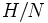# Weakly image-closed characteristic subgroup

Jump to: navigation, search
BEWARE! This term is nonstandard and is being used locally within the wiki. [SHOW MORE]
This article defines a subgroup property: a property that can be evaluated to true/false given a group and a subgroup thereof, invariant under subgroup equivalence. View a complete list of subgroup properties[SHOW MORE]

## Definition

### Definition with symbols

A subgroup$H$ of a group$G$ is termed a weakly image-closed characteristic subgroup of$G$ if, for any normal subgroup$N$ of$G$ contained in$H$, the quotient group$H/N$ is a characteristic subgroup of the quotient group$G/N$.

## Formalisms

### In terms of the weak image condition operator

This property is obtained by applying the weak image condition operator to the property: characteristic subgroup
View other properties obtained by applying the weak image condition operator